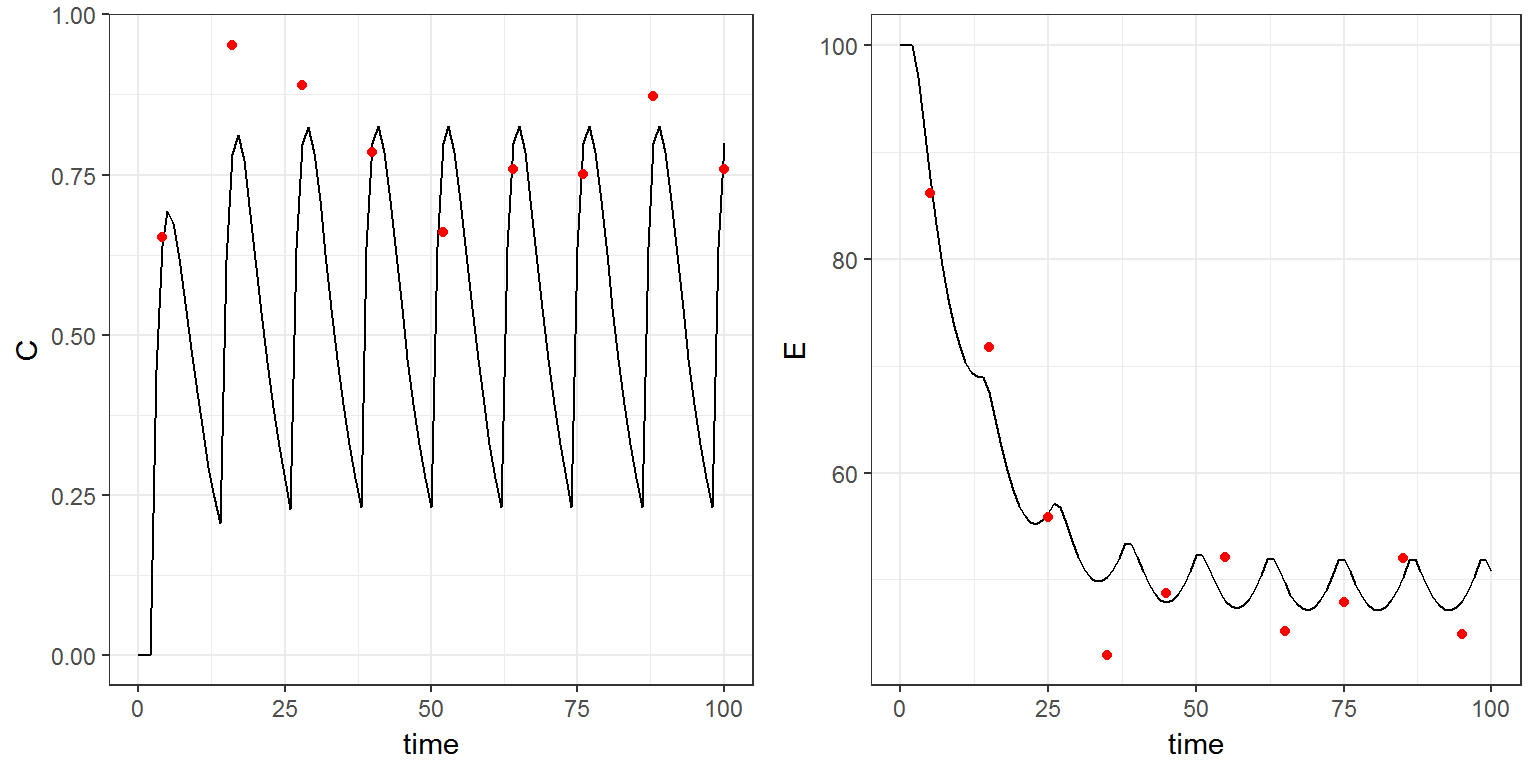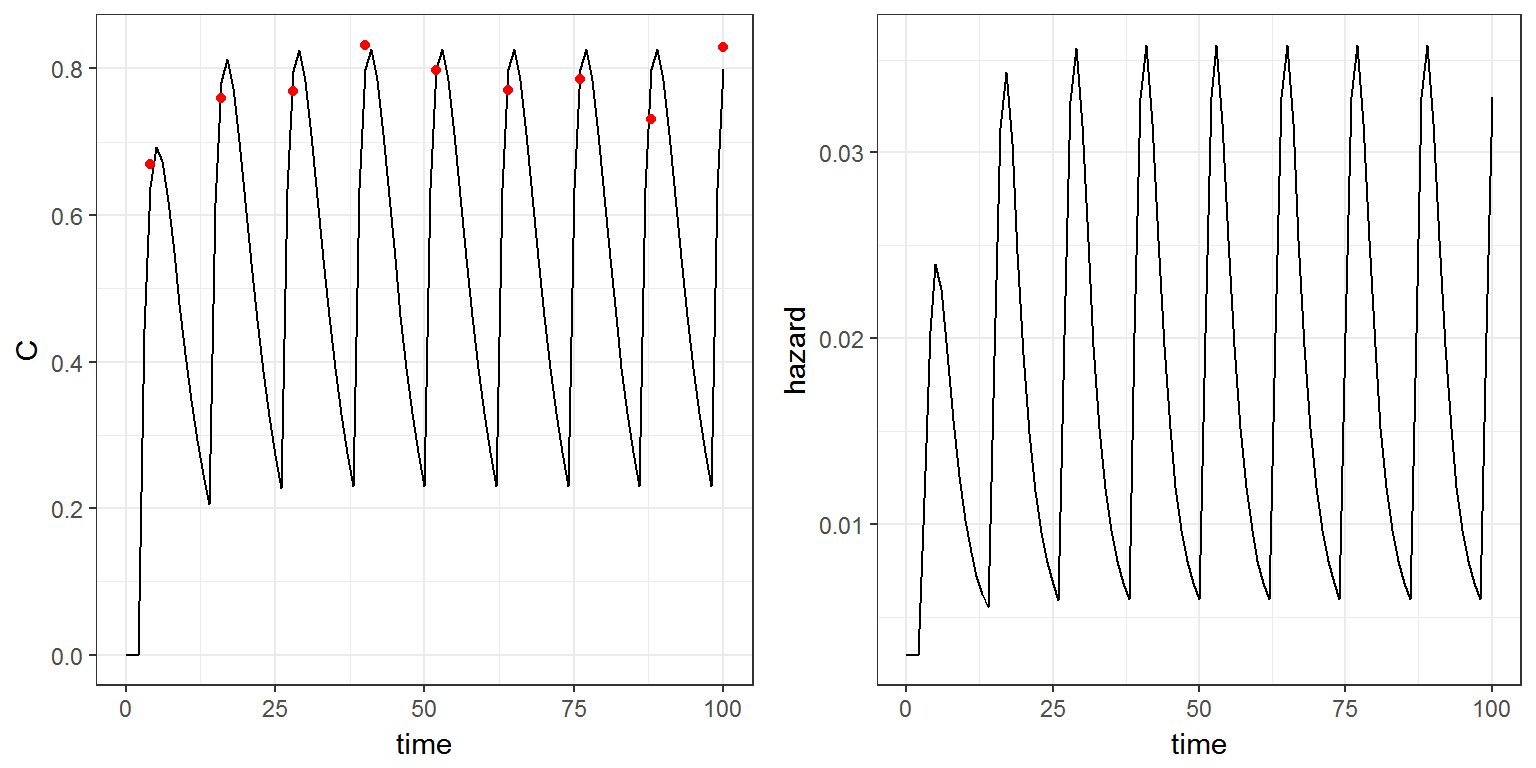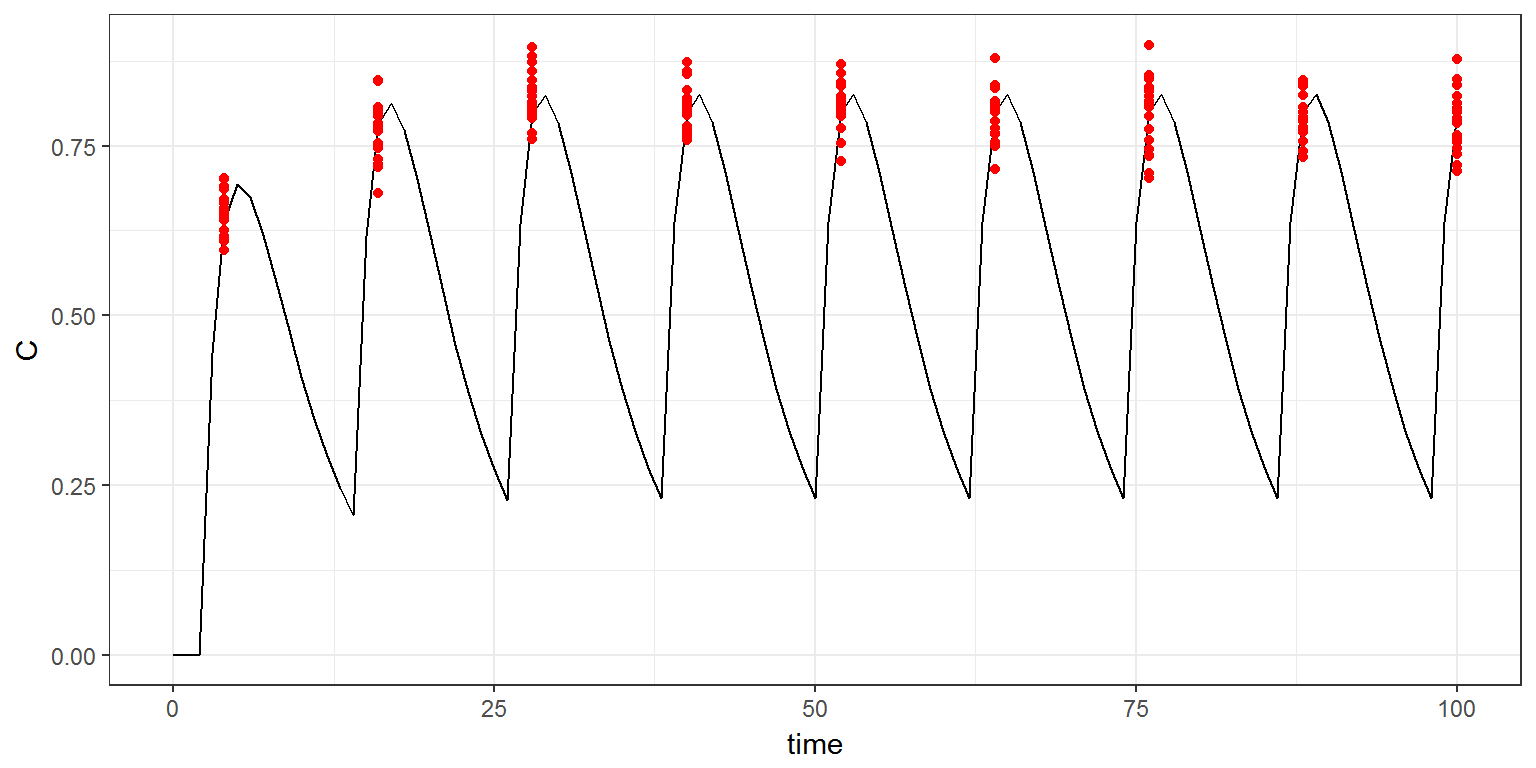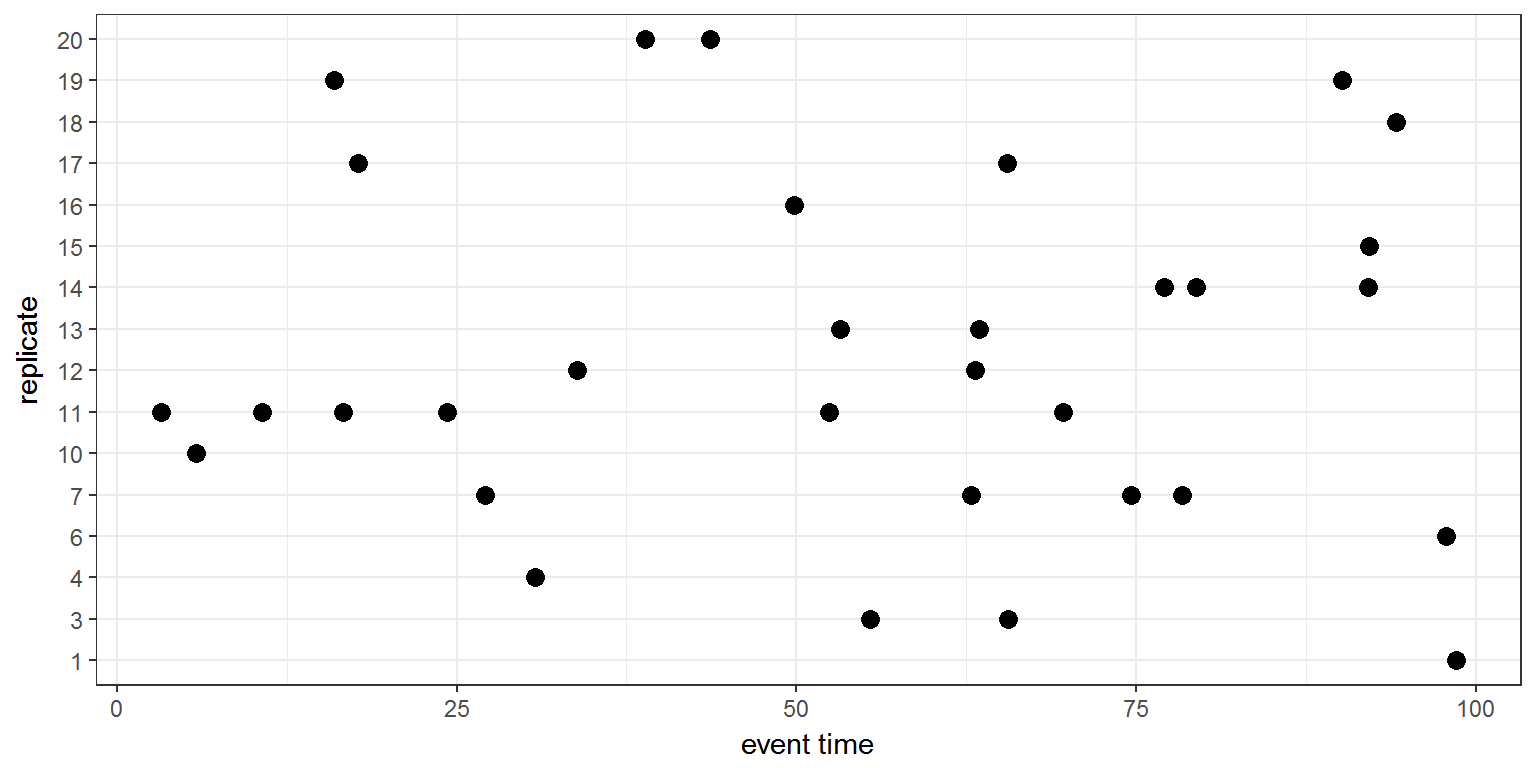R scripts: joint1.R ; joint2.R

$$\newcommand{\esp}{\mathbb{E}\left(#1\right)} \newcommand{\var}{\mbox{Var}\left(#1\right)} \newcommand{\deriv}{\dot{#1}(t)} \newcommand{\prob}{ \mathbb{P}\!(#1)} \newcommand{\eqdef}{\mathop{=}\limits^{\mathrm{def}}} \newcommand{\by}{\boldsymbol{y}} \newcommand{\bc}{\boldsymbol{c}} \newcommand{\bpsi}{\boldsymbol{\psi}} \def\pmacro{\texttt{p}} \def\like{{\cal L}} \def\llike{{\cal LL}} \def\logit{{\rm logit}} \def\probit{{\rm probit}} \def\one{{\rm 1\!I}} \def\iid{\mathop{\sim}_{\rm i.i.d.}} \def\simh0{\mathop{\sim}_{H_0}} \def\df{\texttt{df}} \def\res{e} \def\xomega{x} \newcommand{\argmin}{{\rm arg}\min_{#1}} \newcommand{\argmax}{{\rm arg}\max_{#1}} \newcommand{\Rset}{\mbox{\mathbb{R}}} \def\param{\theta} \def\setparam{\Theta} \def\xnew{x_{\rm new}} \def\fnew{f_{\rm new}} \def\ynew{y_{\rm new}} \def\nnew{n_{\rm new}} \def\enew{e_{\rm new}} \def\Xnew{X_{\rm new}} \def\hfnew{\widehat{\fnew}} \def\degree{m} \def\nbeta{d} \newcommand{\limite}{\mathop{\longrightarrow}\limits_{#1}} \def\ka{k{a}} \def\ska{k{\scriptscriptstyle a}} \def\kel{k{e}} \def\skel{k{\scriptscriptstyle e}} \def\cl{C{\small l}} \def\Tlag{T\hspace{-0.1em}{lag}} \def\sTlag{T\hspace{-0.07em}{\scriptscriptstyle lag}} \def\Tk{T\hspace{-0.1em}{k0}} \def\sTk{T\hspace{-0.07em}{\scriptscriptstyle k0}} \def\thalf{t{1/2}} \newcommand{\Dphi}{\partial_\pphi #1} \def\asigma{a} \def\pphi{\psi} \newcommand{\stheta}{{\theta^\star}} \newcommand{\htheta}{{\widehat{\theta}}}$$

# 1 Introduction

A joint model is one that allows us to simultaneously describe the distribution of different types of observations made on the same individual.

Suppose that we have $$L$$ different types of observation: $$y^{(1)}=(y_{j}^{(1)},1\leq j \leq n_{1})$$, $$y^{(2)}=(y_{j}^{(2)},1\leq j \leq n_{2})$$, …, $$y^{(L)}=(y_{j}^{(L)},1\leq j \leq n_{L})$$, where $$n_{\ell}$$ is the number of observations of type $$\ell$$.

Note that the numbers of observations $$(n_{\ell})$$ and the observation times $$(t_{j}^{(\ell)})$$ may be different.

The joint model is the joint distribution of $$y = (y^{(1)}, y^{(2)}, \ldots, y^{(L)}).$$

This joint distribution is defined in the block DEFINITIONof the Section [LONGITUDINAL].

# 2 Examples

## 2.1 Joint continuous PKPD model

We consider in this example a joint model for continuous PK and PD data. We assume here that the predicted effect $$E$$ is function of the predicted concentration $$C$$:

\begin{align} E(t) &= k_{\rm in}/k_{\rm out} \quad \text{for} \ t\leq 0 \\ \deriv{E} &= k_{\rm in}\left(1 - \frac{C(t)}{IC_{50}+C(t)}\right) - k_{\rm out}E(t) \quad \text{for} \ t\geq 0 \end{align}

We then assume that the measured concentrations $$(y_{j}^{(1)})$$ are log-normally distributed and the measured effects $$(y_{j}^{(2)})$$ normally distributed:

\begin{align} \log(y_{j}^{(1)}) &= \log(C(t_{j}^{(1)})) + a_1 \varepsilon_{j}^{(1)}, \quad ; \quad 1 \leq j \leq n_1 \\ y_{j}^{(2)} &= f_2(t_{j}^{(2)}) + a_2 \varepsilon_{j}^{(2)}, \quad ; \quad 1 \leq j \leq n_2 \end{align}

This model is implemented in joint1.R:

joint.model1 <- inlineModel("
[LONGITUDINAL]
input = {ka, V, Cl, IC50, kin, kout, a1, a2}

EQUATION:
C     = pkmodel(ka, V, Cl)
t0    = 0
E_0   = kin/kout
ddt_E = kin*(1 - C/(IC50+C)) - kout*E

DEFINITION:
Concentration = {distribution = lognormal,
prediction   = C,
sd           = a1}

Effect = {distribution = normal,
prediction   = E,
sd           = a2}
")

We use simulx for computing $$C$$ and $$E$$ every hour between 0h and 100h and for generating measured concentrations every 12 hours between time 4h and 100h and measured effects every 10 hours between time 5h and 100h.

A dose of 10 mg is administrated orally every 12 hours, starting at time 2h.

p <- c(ka=0.5, V=8, Cl=1.5, IC50=0.5, kin=10, kout=0.1, a1=0.1, a2=5)

a <- list(amount = 10, time = seq(2,120,by=12))

f <- list(name = c('C', 'E'),     time = seq(0,100,by=1))
c <- list(name = 'Concentration', time = seq(4,100,by=12))
e <- list(name = 'Effect',        time = seq(5,100,by=10))

res <- simulx(model     = 'model/joint1.txt',
treatment = a,
parameter = p,
output    = list(f, c, e),
settings  = list(seed = 1234))

Here, res is a list with four elements:

names(res)
##  "C"             "E"             "Concentration" "Effect"
##  "treatment"

Let us plot this data:

library("gridExtra")
plot1 = ggplot() + geom_line(data=res$C, aes(x=time, y=C)) + geom_point(data=res$Concentration, aes(x=time, y=Concentration),colour="red")
plot2 = ggplot() + geom_line(data=res$E, aes(x=time, y=E)) + geom_point(data=res$Effect, aes(x=time, y=Effect),colour="red")
grid.arrange(plot1, plot2, ncol=2)## 2.2 Joint PK and time-to-event data model

We consider now a joint model for continuous PK data and repeated events. The hazard $$h$$ is function of the predicted concentration $$C$$: $h(t) = u\, e^{v\,C(t)}$

We also assume that the events are exactly observed until time 100h (right censoring time) This model is implemented in joint2.R:

joint.model2 <- inlineModel("
[LONGITUDINAL]
input = {ka, V, Cl, u, v, a1}

EQUATION:
C = pkmodel(ka, V, Cl)
h = u*exp(v*C)

DEFINITION:
Concentration = {distribution = lognormal,
prediction   = C,
sd           = a1}

Hemorrhaging  = {type               = event,
rightCensoringTime = 100,
hazard             = h}
")

We use Simulx for computing $$C$$ and $$h$$ every hour between 0h and 100h and for generating measured concentrations every 12 hours between time 4h and 100h and repeated events, starting at time 0.

p <- c(ka=0.5, V=8, Cl=1.5, u=0.003, v=3, a1=0.05)

a <- list(amount = 10, time = seq(2,120,by=12))

f <- list(name = c('C', 'h'),     time = seq(0,100,by=1))
c <- list(name = 'Concentration', time = seq(4,100,by=12))
e <- list(name = 'Hemorrhaging',  time = 0)

res1 <- simulx(model     = joint.model2,
treatment = a,
parameter = p,
output    = list(f, c, e),
settings  = list(seed = 12345))

Let us see the simulated events:

print(res1$Hemorrhaging) ## time Hemorrhaging ## 1 0.00000 0 ## 2 86.95924 1 ## 3 99.83272 1 ## 4 100.00000 0 Indeed, in this example, • the experiment starts at time 0, which means that the first event will occur after time 0 • there is one observed event at time 86.96h • there is one observed event at time 99.83h • the next event will occur after time 100 We can also plot the predicted and observed concentrations and the hazard function plot1 = ggplot() + geom_line(data=res1$C, aes(x=time, y=C)) +
geom_point(data=res1$Concentration, aes(x=time, y=Concentration),colour="red") plot2 = ggplot() + geom_line(data=res1$h, aes(x=time, y=h)) +
ylab("hazard")  + theme(legend.position="none")
grid.arrange(plot1, plot2, ncol=2)Instead of simulating data for a single individual, we can simulate data for $$N=20$$ individuals with the same model, using the input argument group

N   <- 20
res2 <- simulx(model     = joint.model2,
treatment = a,
parameter = p,
output    = list(f, c, e),
group     = list(size = N, level='longitudinal'),
settings  = list(seed = 121212))

We can plot the predicted and observed concentrations

plot3 = ggplot() + geom_line(data=res2$C, aes(x=time, y=C, group=id)) + geom_point(data=res2$Concentration, aes(x=time, y=Concentration),colour="red")
plot(plot3)We can also plot the observed events for the 20 individuals:

h1    = res2$Hemorrhaging[res2$Hemorrhaging[,3]==1,]
plot4 = ggplot()+geom_point(data=h1, aes(x=time,y=id), size=3) +
xlab("event time") + ylab("replicate")  + theme(legend.position="none")
plot(plot4)